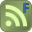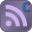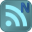Home

Top - XLC

How to present Calculations in Excel

This video is best viewed in full screen mode. Press play and double click the screen to maximise.

DiscussionHow to present Calculations in Excel - JohnDoyle[Admin] May 23rd 2008 4:00This thread is a discussion about How to present Calculations in Excel video.This tutorial shows you why it's a good idea to present calculations using the XLC template that can be downloaded from the ExcelCalcs.com site. If you are not familiar with XLC it's a free addin from ExcelCalcs.com and it displays you cell formulae as mathematical notation like these ones here. Login to write comment

Date :02 May 2008
Views :7819
Votes :0 Rating :0

This tutorial shows you why it's a good idea to present calculations using the XLC template that can be downloaded from the ExcelCalcs.com site.
Tags:
 Embed:

Sort ByVideos in "XLC" category1) XLC Tutorial Ok so you have installed XLC now lets get up and running. This video explain what to do when you start up Excel for the first time with the XLC addin loaded. Topics covered: Name and show equations; ...2) XLC Tutorial Algebraic and numeric equation displays; Equation cloning; Behaviour of EQS() functions.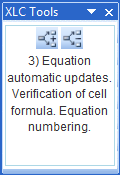3) XLC Tutorial Equation automatic updates; Verification of cell formula; Equation numbering.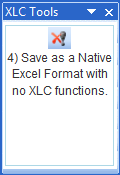4) XLC Tutorial Save as a Native Excel File with no XLC functions.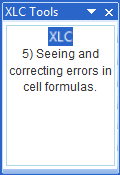5) XLC Tutorial Seeing and correcting errors in cell formulas.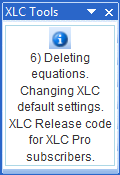6) XLC Tutorial Deleting equations; Changing XLC default settings; XLC Release code for XLC Pro subscribers.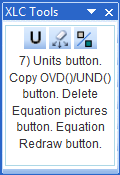7) XLC Tutorial Units button; Copy OVD()/UND() button; Delete Equation pictures button; Equation Redraw button.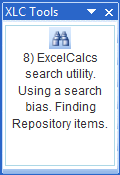8) XLC Tutorial ExcelCalcs search utility; Using a search bias; Finding Repository items.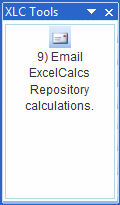9) XLC Tutorial Email ExcelCalcs Repository calculations.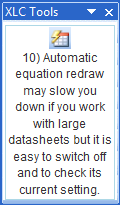10) XLC Tutoria... How to switch of the audomatic equation redraw and naming features of XLC the addin for Excel.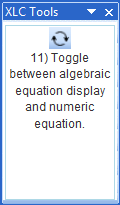11) XLC Tutoria... How to switch between algebraic eqation display and numeric equation display with XLC the addin for Excel..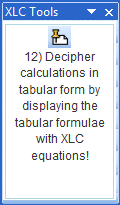12) XLC Tutoria... How to make equations from tabular data with XLC the addin for Excel.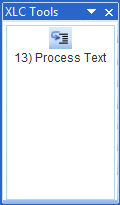13) XLC Tutoria... An XLC tool to help us format cells, textboxes and charts with mathematical notation.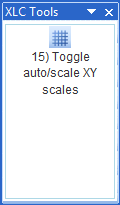15) XLC Tutoria... Learn how to automatically adjust scatter chart X and Y scales so that sketches are correctly scaled.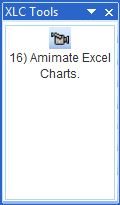16) XLC Tutoria... This is best viewed in full screen model. Double click the player.Introducing XLC... A quick guide around the new features in XLC v10_09 including making equations from tabular data, algebra/number equation toggle and easy switch of the XLC audomatic redraw features.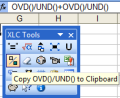Copy OVD()/UND(... A simple tool to save time typing XLC OVD()/UND() functions.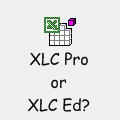XLC Pro or XLC ... What is the difference?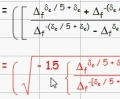Enhanced Equati... This is best viewed in full screen model. Double click the player.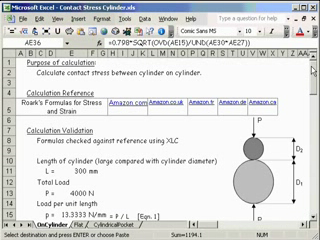How to present ... This tutorial shows you why it's a good idea to present calculations using the XLC template that can be downloaded from the ExcelCalcs.com site.

 Jump to: / 1We have 10 guests and 36 members online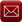Email (preferred method of contact)US +1 617 5008224EU +44 113 8152220